The UNIVARIATE Procedure
 WEIGHT Statement

WEIGHT variable ;

The WEIGHT statement specifies numeric weights for analysis variables in the statistical calculations. The UNIVARIATE procedure uses the values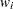of the WEIGHT variable to modify the computation of a number of summary statistics by assuming that the variance of theth value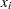of the analysis variable is equal to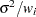, where
is an unknown parameter. The values of the WEIGHT variable do not have to be integers and are typically positive. By default, observations with nonpositive or missing values of the WEIGHT variable are handled as follows:1

• If the value is zero, the observation is counted in the total number of observations.

• If the value is negative, it is converted to zero, and the observation is counted in the total number of observations.

• If the value is missing, the observation is excluded from the analysis.

To exclude observations that contain negative and zero weights from the analysis, use EXCLNPWGT. Note that most SAS/STAT procedures, such as PROC GLM, exclude negative and zero weights by default. The weight variable does not change how the procedure determines the range, mode, extreme values, extreme observations, or number of missing values. When you specify a WEIGHT statement, the procedure also computes a weighted standard error and a weighted version of Student’stest. The Student’stest is the only test of location that PROC UNIVARIATE computes when you weight the analysis variables.

When you specify a WEIGHT variable, the procedure uses its values,, to compute weighted versions of the statistics2 provided in the Moments table. For example, the procedure computes a weighted mean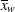and a weighted variance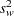as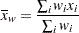and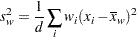whereis theth variable value. The divisor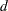is controlled by the VARDEF= option in the PROC UNIVARIATE statement.

The WEIGHT statement does not affect the determination of the mode, extreme values, extreme observations, or the number of missing values of the analysis variables. However, the weightsare used to compute weighted percentiles.3 The WEIGHT variable has no effect on graphical displays produced with the plot statements.

The CIPCTLDF, CIPCTLNORMAL, LOCCOUNT, NORMAL, ROBUSTSCALE, TRIMMED=, and WINSORIZED= options are not available with the WEIGHT statement.

To compute weighted skewness or kurtosis, use VARDEF=DF or VARDEF=N in the PROC statement.

You cannot specify the HISTOGRAM, PROBPLOT, or QQPLOT statements with the WEIGHT statement.

When you use the WEIGHT statement, consider which value of the VARDEF= option is appropriate. See VARDEF= and the calculation of weighted statistics for more information.Previous Page | Next Page | Top of Page